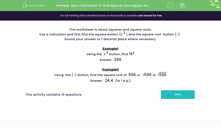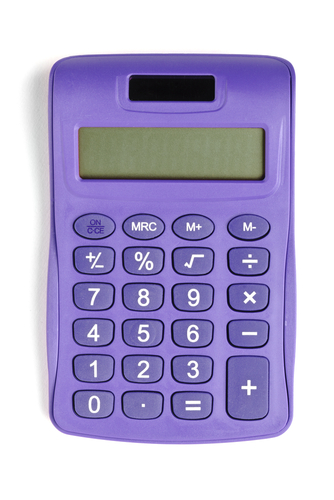# Use a Calculator to Find Squares and Square Roots

In this worksheet, students will use a calculator to find the squares and square roots of the given numbers.Key stage:  KS 3

Curriculum topic:   Number

Curriculum subtopic:   Use Calculators/Technology for Accuracy

Popular topics:   Numbers worksheets

Difficulty level:#### Worksheet Overview

This activity is about squares and square roots.

We're going to practise using a calculator and finding the square button (x2 ) and the square root button (√)Example 1

Using the  x2 button, find 162

First, put in 16, then x2, and finally =

Example 2

Using  the (√) button, find the square root of  596 or  √596

First, tap the √ button, then 596, and finally =

The answer is 24.4 to one decimal place.

Let's give it a try! If you need to remind yourself of the order of the buttons, just click on the red button and you'll be able to see this introduction again.### What is EdPlace?

We're your National Curriculum aligned online education content provider helping each child succeed in English, maths and science from year 1 to GCSE. With an EdPlace account you’ll be able to track and measure progress, helping each child achieve their best. We build confidence and attainment by personalising each child’s learning at a level that suits them.

Get started